# WO2017037836A1 - Signal transmission apparatus and signal transmission system - Google Patents

## Info

Publication number
WO2017037836A1
WO2017037836A1 PCT/JP2015/074692 JP2015074692W WO2017037836A1 WO 2017037836 A1 WO2017037836 A1 WO 2017037836A1 JP 2015074692 W JP2015074692 W JP 2015074692W WO 2017037836 A1 WO2017037836 A1 WO 2017037836A1
Authority
WO
WIPO (PCT)
Prior art keywords
signal
data
filter coefficient
detection
logical data
Prior art date
Application number
PCT/JP2015/074692
Other languages
French (fr)
Japanese (ja)
Inventor

Original Assignee

Priority date (The priority date is an assumption and is not a legal conclusion. Google has not performed a legal analysis and makes no representation as to the accuracy of the date listed.)
Filing date
Publication date
Application filed by 株式会社日立製作所 filed Critical 株式会社日立製作所
Priority to PCT/JP2015/074692 priority Critical patent/WO2017037836A1/en
Publication of WO2017037836A1 publication Critical patent/WO2017037836A1/en

• 238000004364 calculation methods Methods 0 abstract claims description 35
• 238000004422 calculation algorithm Methods 0 abstract claims description 9
• 230000003044 adaptive Effects 0 abstract claims description 7
• 230000001702 transmitter Effects 0 claims description 16
• 230000000875 corresponding Effects 0 claims description 10
• 101700000816 CTR1 family Proteins 0 abstract description 3
• 101700077314 PDX1 family Proteins 0 abstract description 3
• 102100014615 SLC7A1 Human genes 0 abstract description 3
• 239000000758 substrates Substances 0 description 20
• 239000004065 semiconductor Substances 0 description 14
• 238000004891 communication Methods 0 description 9
• 230000000694 effects Effects 0 description 7
• 238000000034 methods Methods 0 description 7
• 230000001965 increased Effects 0 description 6
• 230000002829 reduced Effects 0 description 6
• 230000001603 reducing Effects 0 description 6
• 238000006011 modification Methods 0 description 5
• 230000004048 modification Effects 0 description 5
• 238000006722 reduction reaction Methods 0 description 5
• 210000001508 Eye Anatomy 0 description 4
• 230000001419 dependent Effects 0 description 3
• 230000004044 response Effects 0 description 3
• 230000000295 complement Effects 0 description 2
• 230000002596 correlated Effects 0 description 2
• 230000001934 delay Effects 0 description 2
• 230000003111 delayed Effects 0 description 2
• 238000005516 engineering processes Methods 0 description 2
• 239000011133 lead Substances 0 description 2
• 230000003321 amplification Effects 0 description 1
• 230000002238 attenuated Effects 0 description 1
• 239000008264 clouds Substances 0 description 1
• 238000007906 compression Methods 0 description 1
• 238000002592 echocardiography Methods 0 description 1
• 239000008072 ether Substances 0 description 1
• 239000000284 extracts Substances 0 description 1
• 230000035611 feeding Effects 0 description 1
• 238000001914 filtration Methods 0 description 1
• 230000015654 memory Effects 0 description 1
• 239000000203 mixtures Substances 0 description 1
• 238000003199 nucleic acid amplification method Methods 0 description 1
• 230000002250 progressing Effects 0 description 1
• 230000003252 repetitive Effects 0 description 1
• 238000004088 simulation Methods 0 description 1
• 230000000576 supplementary Effects 0 description 1
• 230000001629 suppression Effects 0 description 1

## Images

•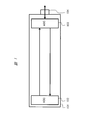•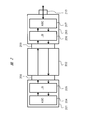•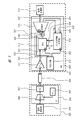•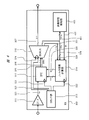•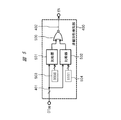•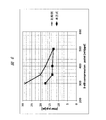•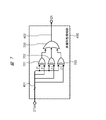•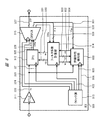•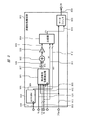•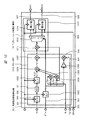••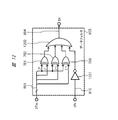•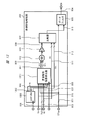•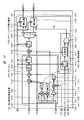•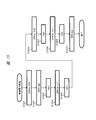•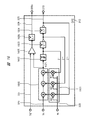## Classifications

• HELECTRICITY
• H04ELECTRIC COMMUNICATION TECHNIQUE
• H04BTRANSMISSION
• H04B3/00Line transmission systems
• H04B3/02Details
• H04B3/04Control of transmission; Equalising
• H04B3/06Control of transmission; Equalising by the transmitted signal

## Abstract

A continuous time linear equalizer (CTLE) 311 outputs a second signal that has been waveform-equalized by amplifying the higher frequency side of an input first signal. A decision feedback equalizer (DFE) 312 waveform-equalizes the input second signal by use of a filter factor (Wi) and makes a logic decision on the waveform-equalized second signal to thereby output logic data of the second signal (DTS), and further, the DFE 312 detects a difference between a peak value of the waveform-equalized signal and a target peak value (TGT) to thereby output a target value error (ERRs). A filter factor calculation unit 314 receives the logic data and target value error from the DFE 312 and calculates a filter factor (Wi) of the DFE 312 by use of an adaptive algorithm. A nonlinearity detection unit 400 monitors the logic data from the DFE 312, detects a data pattern of n bits (where n is an integer equal to or greater than two) successively having the same logic level, and instructs the filter factor calculation unit 314 not to perform the filter factor calculation for the detected data pattern.

## Description

Signal transmission device and signal transmission system

The present invention relates to a signal transmission device and a signal transmission system, for example, a technique for automatically converging the equalization amount of an equalizer in a receiver for high-speed wired transmission.

In recent years, the amount of communication has been increasing due to the spread of streaming and the spread of cloud services, and in response to this, the increase in the amount of data in information devices and the improvement of processing capacity are progressing. At that time, the communication speed inside and outside the apparatus, which is a bottleneck for large-scale data processing, is also improving. For example, in communication outside the apparatus, support for standards such as 100G Ether and 32GFC is progressing, and speeding up is progressing to communication speeds of 25 Gbps and 28 Gbps per lane.

With the increase in communication speed, for example, the loss of the substrate is increased, a loss of 35 dB or more is expected at 14 GHz, and the influence of reflection due to impedance mismatch cannot be ignored (see Non-Patent Document 1). For this reason, it is necessary to suppress jitter as much as possible, and a technique for suppressing an increase in jitter by adapting the filter constant of the equalizer to environmental fluctuations is important. Conventionally, there is a technique for outputting a response stored in a memory according to an input data pattern and equalizing it, and a technique for adapting the response pattern according to an environmental change has been proposed (see Non-Patent Document 2). ).

John F. Bulzacchelli and et. Al., "A 28-Gb / s 4-Tap FFE / 15-Tap DFE Serial Link Transceiver in 32-nm SOI CMOS Technology", JSSC, Vol.47, No.12, December 2012 Nils Holte and Steinar Stueflotten, "A New Digital Echo Canceler for Two-Wire Subscriber Lines," IEEE Transactions on Communications, Vol. COM-29, No.11, November 1981

Acceleration of communication has increased the path loss on the board and cable, and the signal amplitude difference tends to increase at low and high frequencies. Thereby, the amplitude difference of the input signal of the receiver increases in accordance with the data pattern of the transmitter. On the other hand, in order to secure SNR (Signal to Noise Ratio), the amplitude of the transmission signal of the transmitter must be increased. As a result, an amplifier that amplifies the input signal of the receiver is required to perform linear amplification in a wide input / output range (that is, to achieve high linearity). However, in order to cope with high-speed operation, for example, when a fine CMOS (Complementary Metal-Oxide-Semiconductor) process is used, it is necessary to lower the power supply voltage. If so, it may be difficult to achieve high linearity of the amplifier.

If high linearity cannot be realized, the output signal from the amplifier will contain both non-linear components and ISI (Inter Symbol Interference) components. These two components both depend on the data pattern, but have different functions because they are generated by different mechanisms. As described above, it is not easy to separate two components having a correlation and different functions. On the other hand, the output signal from the amplifier is equalized by an equalizer. The equalizer automatically adjusts the filter coefficient according to environmental fluctuations in order to reduce the ISI component. However, since the above-mentioned two components are mixed in the output signal from the amplifier, there may be a case where an error when the filter coefficient is automatically adjusted by the equalizer is increased. In this case, the convergence value of the filter coefficient is deviated from the ideal value, and a necessary equalization amount cannot be obtained (that is, the ISI component cannot be reduced), and the jitter may increase.

The present invention has been made in view of such circumstances, and one of its purposes is to provide a signal transmission device and a signal transmission system capable of realizing jitter reduction.

The above and other objects and novel features of the present invention will become apparent from the description of the present specification and the accompanying drawings.

Among the inventions disclosed in the present application, the outline of a typical embodiment will be briefly described as follows.

The signal transmission apparatus according to the present embodiment includes a continuous-time linear equalizer, a decision feedback equalizer, a filter coefficient calculation unit, and a nonlinearity detection unit. The continuous time linear equalizer outputs a second signal subjected to waveform equalization by amplifying the high frequency side of the input first signal. The decision feedback equalizer equalizes the waveform of the input second signal using a filter coefficient, outputs the logical data of the second signal by logically determining the waveform, and the peak value of the waveform equalized signal The target value error is output by detecting the difference between the target value and the target peak value. The filter coefficient calculation unit receives the logical data and the target value error from the decision feedback equalizer, and calculates the filter coefficient of the decision feedback equalizer using an adaptive algorithm. The non-linearity detection unit monitors the logical data from the decision feedback equalizer, detects a data pattern in which n bits (n is an integer of 2 or more) are continuously at the same logical level, and outputs the data pattern to the filter coefficient calculation unit. And instructing not to calculate a filter coefficient for the detected data pattern.

A brief description of the effect obtained by the representative embodiment of the invention disclosed in the present application makes it possible to reduce jitter.

1 is a schematic diagram illustrating a configuration example of a signal transmission system according to a first embodiment of the present invention. It is the schematic which shows the other structural example in the signal transmission system by Embodiment 1 of this invention. FIG. 3 is a block diagram illustrating a configuration example of each signal transmission device studied as a premise of the first embodiment in the signal transmission system of FIGS. 1 and 2. FIG. 3 is a block diagram illustrating a configuration example of a main part of the signal transmission device according to the first embodiment in the signal transmission system of FIGS. 1 and 2; It is a circuit diagram which shows the structural example of the nonlinearity detection part in FIG. It is explanatory drawing which shows an example of the effect at the time of using the receiver of FIG. 4 and FIG. It is a circuit diagram which shows another structural example of the nonlinearity detection part in FIG. In the signal transmission apparatus according to the second embodiment, FIG. It is a block diagram which shows the structural example of the nonlinearity detection part in FIG. It is a circuit diagram which shows the structural example of the coefficient detector classified by frequency in FIG. FIG. 10 is a circuit diagram illustrating a configuration example of a data filter in FIG. 9. FIG. 10 is a circuit diagram illustrating another configuration example of the data filter in FIG. 9. In the signal transmission apparatus according to the third embodiment, FIG. It is a circuit diagram which shows the structural example of the coefficient detector classified by frequency in FIG. It is a flowchart which shows an example of the operation | movement sequence of the controller in FIG. It is the schematic which shows the typical structural example of DFE in FIG.

In the following embodiment, when it is necessary for the sake of convenience, the description will be divided into a plurality of sections or embodiments. However, unless otherwise specified, they are not irrelevant, and one is the other. Some or all of the modifications, details, supplementary explanations, and the like are related. Further, in the following embodiments, when referring to the number of elements (including the number, numerical value, quantity, range, etc.), especially when clearly indicated and when clearly limited to a specific number in principle, etc. Except, it is not limited to the specific number, and may be more or less than the specific number.

Further, in the following embodiments, the constituent elements (including element steps and the like) are not necessarily indispensable unless otherwise specified and apparently essential in principle. Needless to say. Similarly, in the following embodiments, when referring to the shapes, positional relationships, etc. of the components, etc., the shapes are substantially the same unless otherwise specified, or otherwise apparent in principle. And the like are included. The same applies to the above numerical values and ranges.

The circuit elements constituting each functional block of the embodiment are not particularly limited, but are formed on a semiconductor substrate such as single crystal silicon by a known integrated circuit technology such as a CMOS (complementary MOS transistor). .

Hereinafter, embodiments of the present invention will be described in detail with reference to the drawings. Note that components having the same function are denoted by the same reference symbols throughout the drawings for describing the embodiment, and the repetitive description thereof will be omitted.

(Embodiment 1)
<< Schematic configuration of signal transmission system >>
FIG. 1 is a schematic diagram showing a configuration example of a signal transmission system according to Embodiment 1 of the present invention. FIG. 1 shows a configuration example of a signal transmission system that performs wired transmission on the same substrate. The substrate 101 includes a signal processing ASIC (Application Specific Integrated Circuit) 102 having a waveform equalization function, a communication ASIC 103 having a waveform equalization function, and a connector 104. The ASIC 102 and the ASIC 103 communicate with each other via a wiring on the substrate 101, and the ASIC 103 communicates with another device through the connector 104.

FIG. 2 is a schematic diagram showing another configuration example of the signal transmission system according to Embodiment 1 of the present invention. FIG. 2 shows a configuration example of a signal transmission system in which the communication in FIG. 1 is performed not only on the same board but also on different boards and a repeater is mounted. The substrate 201 mounts the signal processing ASIC 204, the repeater 205, and the connector 208, and the substrate 203 mounts the signal processing ASIC 207, the repeater 206, and the connectors 209 and 210. The substrate 202 is connected to the substrates 201 and 203 via connectors 208 and 209, respectively.

As in the signal flow in FIG. 1, the signal processing ASIC 204 communicates with another device connected to the end of the connector 210 through the communication ASIC 207. At this time, the signal output from the ASIC 204 is relayed to the board 202 by the relay 205 and transmitted to the relay 206 via the wiring on the board 202. The repeater 206 relays the transmitted signal to the communication ASIC 207, and the ASIC 207 transmits the relayed signal to an external device through the connector 210. Conversely, a signal received by the ASIC 207 from the external device through the connector 210 is received by the ASIC 204 via the repeater 206, the substrate 202, and the repeater 205 in this order.

1 and 2, each of the ASICs 102, 103, 204, 207 and the repeaters 205, 206 is a signal transmission device in which a transmitter and a receiver are mounted on one semiconductor chip, for example. In FIG. 2, for example, the substrate 202 is a backplane or the like, and each of the substrates 201 and 203 is a card or the like that can be mounted on the backplane.

<< Prerequisite configuration of signal transmission equipment >>
FIG. 3 is a block diagram illustrating a configuration example of each signal transmission device studied as a premise of the first embodiment in the signal transmission system of FIGS. 1 and 2. As an example, the semiconductor chips (signal transmission devices) 301 and 303 in FIG. 3 correspond to the ASICs 102 and 103 in FIG. 1, respectively, and the transmission path 302 in FIG. 3 corresponds to the wiring on the substrate 101 in FIG. As another example, the semiconductor chips 301 and 303 in FIG. 3 correspond to the repeaters 205 and 206 in FIG. 2, respectively, and the transmission path 302 in FIG. 3 corresponds to the wiring on the substrate 202 in FIG.

The semiconductor chip (signal transmission device) 301 includes a transmitter 304 and a signal processing unit 305, and the semiconductor chip (signal transmission device) 303 includes a receiver 309 and a signal processing unit 310. A signal transmitted from the transmitter 304 of the semiconductor chip 301 is transmitted through the transmission path 302 and received by the receiver 309 of the semiconductor chip 303. Here, each of the semiconductor chips 301 and 304 is configured to include one of a transmitter and a receiver for convenience, but actually includes both a transmitter and a receiver.

The transmitter 304 includes a feed forward equalizer (hereinafter referred to as FFE) 307, a multiplexer 306, and a PLL (Phase Locked） Loop) 308. The receiver 309 includes a continuous time linear equalizer (hereinafter referred to as CTLE (Continuous Time Linear Equalizer)) 311, a decision feedback equalizer (hereinafter referred to as DFE (Decision Feedback Equalizer)) 312, and a clock source. 313, a filter coefficient calculation unit 314, and a demultiplexer 315.

Next, the operation of FIG. 3 will be described. First, the signal processing unit 305 performs predetermined signal processing and transmits a parallel data signal to the transmitter 304 as necessary. The multiplexer (parallel-serial converter) 306 receives the parallel data signal and converts the parallel data signal into a serial data signal in synchronization with the clock from the PLL 308. The FFE 307 receives the serial data signal, processes the data signal into a signal that emphasizes the high frequency side in advance, and transmits the signal to the transmission path 302. Specifically, the FFE 307 performs waveform equalization so as to reduce the gain on the low frequency side, for example.

The data signal 315 that has passed through the transmission path 302 is received by the receiver 309 in the semiconductor chip 303. At this time, the data signal transmitted from the transmitter 304 is attenuated through the transmission path 302. The CTLE 311 performs waveform equalization by amplifying the high-frequency side of the received data signal, and outputs the signal (Vo) 320 on which waveform equalization has been performed to the DFE 312. The DFE 312 performs waveform equalization on the signal (Vo) 320 such that the low frequency side is attenuated and the high frequency side is amplified in accordance with the filter coefficient (Wi) 330 from the filter coefficient calculation unit 314 and amplified. After that, logical determination (positive / negative determination) is performed.

The DFE 312 sequentially latches the logical data (DTS) 325 that is the result of the logical determination (positive / negative determination) in response to the clock 321 from the clock source 313, and outputs it as a serial data signal. Further, the DFE 312 compares not only the logical determination of data but also the filter convergence target value (target peak value) (TGT) 331 from the filter coefficient calculation unit 314 and the peak value of the signal subjected to waveform equalization by the DFE 312. Then, a filter convergence target value error (ERRs) 326 is output.

The demultiplexer (serial / parallel converter) 315 receives the logical data (DTS) 325 and the filter convergence target value error (ERRs) 326, and converts these serial signals into parallel signals using the clocks 323 and 324 from the clock source 313. To do. As a result, the demultiplexer 315 outputs logical data (DTP) 327 as a parallel data signal, logical data (DTp) 328 as a part thereof, and a filter convergence target value error (ERRp) 329 as a parallel signal. To do.

For example, when the clock 323 has a frequency 1/32 that of the clock 324, the demultiplexer 315 outputs a parallel signal every 32 cycles of the clock 324. In this case, the logical data (DTP) 327 is configured by, for example, a 32-bit area, and the logical data (DTp) 328 is configured by a predetermined adjacent multiple-bit area in the 32-bit area. The The number of bits of the plurality of bits is determined based on the number of taps of the DFE 312, for example.

The filter coefficient calculation unit 314 receives the logical data (DTp) 328 and the filter convergence target value error (ERRp) 329. In other words, the logical data (DTS) 325 and the filter convergence target value error (ERRs) 326 from the DFE 312 are input to the filter coefficient calculation unit 314 via the demultiplexer 315. The filter coefficient calculation unit 314 operates with the clock 322 from the clock source 313, receives the logical data (DTp) 328 and the filter convergence target value error (ERRp) 329, and sets the filter coefficient (Wi) 330 of the DFE 312 by an adaptive algorithm. calculate. As an adaptive algorithm, an LMS (Least） Mean Square) algorithm is typically known.

Furthermore, the filter coefficient calculation unit 314 also calculates a filter convergence target value (target peak value) (TGT) 331. The filter convergence target value (target peak value) (TGT) 331 can be, for example, an average value of the signal amplitude after waveform equalization (V (Z 0 ) in FIG. 16 described later). That is, when waveform equalization is performed, the signal amplitude after waveform equalization is ideally constant regardless of the data pattern. Therefore, the filter coefficient calculation unit 314 detects the average value of the signal amplitude and uses it as a target value so that the instantaneous amplitude value approaches the target value (that is, the target value error is minimized). An arithmetic process that converges the filter coefficients may be performed using an adaptive algorithm.

The signal processing unit 310 performs predetermined signal processing using the logical data (DTP) 327. For example, when the semiconductor chip 303 is the repeater 205 in FIG. 2, the signal processing unit 310 performs error correction or the like on the logical data (DTP) 327 and then stores the logical data in the semiconductor chip 303. The relaying process is performed via a transmitter (not shown).

FIG. 16 is a schematic diagram illustrating a schematic configuration example of the DFE in FIG. As shown in FIG. 16, the DFE 312 receives the signal (Vo) 320 from the CTLE 311 and feeds back the multiplication result of the filter coefficient (Wi) 330 and the logical data of the past signal (Vo) 320 to the signal. Waveform equalization. Here, the case of 3 taps is taken as an example, and the multiplier block 1601 outputs each logical data (Z in the previous to third cycles) output from the determiner with latch 1602, the latch circuit 1603, and the latch circuit 1604, respectively. −1 , Z −2 , Z −3 ) and the first to third tap filter coefficients W1 to W3, respectively. The adder block 1600 performs waveform equalization by adding the multiplication result from the multiplier block 1601 to the signal (Vo) 320. This eliminates intersymbol interference (ISI) components.

Further, the DFE 312 outputs the logical data of the signal (Vo) 320 by performing logical determination (positive / negative determination) and latching of the waveform equalized signal (V (Z 0 )) by the determination unit 1602 with latch. The logical data is sequentially transferred to the latch circuit 1603 and the latch circuit 1604 in synchronization with the clock. Further, the DFE 312 detects the difference between the wave height value of the waveform equalized signal and the filter convergence target value (target wave height value) (TGT) 331 by the error detector 1605, so that the filter convergence target value error (ERRs) 326 is detected. Is output after adjusting the timing by the delay circuit 1606.

<< Examples of problems with signal transmission equipment (prerequisite configuration) >>
In the configuration and operation as described above, the CTLE 311 in FIG. 3 is an analog amplifier and has a limited input / output range that can be linearly amplified. In addition, with the use of a fine CMOS process as the speed increases, the power supply voltage decreases, and the input / output range in which linearity can be secured is becoming narrower. For example, in a 28 nm CMOS process, the power supply voltage is 1 V or less, and the input range in which linear operation is possible with a general resistive load type amplifier is only about 200 to 300 mV, for example.

Furthermore, the loss in the transmission path 302 on the substrate tends to increase on the high frequency side, and the signal level difference between the low frequency side and the high frequency side increases as the speed increases. At 28 Gbps, as described in Non-Patent Document 1, a signal level difference of 30 dB or more can occur. On the other hand, since the SNR on the high frequency side is ensured, the transmission amplitude cannot be reduced, and on the low frequency side where the loss in the transmission path 302 is small, the signal level increases to, for example, 500 mV or more, and the linear operation of the CTLE 311 becomes difficult. As a result, the signal (Vo) 320 from the CTLE 311 includes components added to the signal due to the influence of nonlinearity in addition to components added to the signal by ISI.

The component due to ISI depends on the data pattern because it follows the frequency characteristics of the signal path, and the component due to non-linearity depends on the signal level (and hence the frequency of the signal), so it also depends on the data pattern. Both depend on the data pattern, but because the causes are different from each other, the same trend does not occur. For example, the filter coefficient calculation unit 314 obtains a correlation between the data pattern (that is, each bit of the logical data (DTp) 328) and the filter convergence target value error (ERRp) 329, and uses the LMS algorithm or the like. A filter coefficient (Wi) 330 is calculated.

At this time, the filter coefficient calculation unit 314 is difficult to extract only the ISI component from the correlation result, for example, because the data pattern dependency of the component due to ISI and the component due to nonlinearity do not have the same tendency. As a result, an error in the calculation result of the filter coefficient (Wi) 330 (that is, an error with respect to an ideal waveform equalization amount necessary for eliminating the ISI component) may occur. In particular, an error when the filter coefficient (Wi) 330 is automatically adjusted in accordance with environmental fluctuations such as temperature and power supply voltage fluctuations becomes large. As described above, when the waveform equalization amount is deviated, the ISI component cannot be sufficiently eliminated, and there is a possibility that jitter increases (for example, eye reduction in a so-called eye pattern).

<< Configuration of Signal Transmission Device (Embodiment 1) >>
Here, in wired transmission, loss is low at low frequencies and loss is high at high frequencies. Therefore, the influence of the linearity of CTLE 311 is large for signals on the low frequency side and small for signals on the high frequency side. In addition, the effect of nonlinearity is steeper than ISI. In this case, if a signal having a small signal level and a small influence of linearity is used for convergence of the filter coefficient (Wi) 330, it is considered that the influence of the component due to the nonlinearity can be eliminated and only the ISI component can be extracted sufficiently. . Therefore, in the first embodiment, a signal affected by non-linearity is detected, and only a linearly operating signal is used for calculation of the filter coefficient (Wi) 330. Specifically, by not using the low-frequency signal for calculating the filter coefficient (Wi) 330, the influence of nonlinearity is suppressed and the filter coefficient (Wi) 330 is optimally converged.

FIG. 4 is a block diagram illustrating a configuration example of a main part of the signal transmission device according to the first embodiment in the signal transmission system of FIGS. 1 and 2. The signal transmission device according to the first embodiment is configured to include a receiver 309 as in the semiconductor chip (signal transmission device) 303 of FIG. 3. As shown in FIG. The configuration is different from FIG. The receiver 309 illustrated in FIG. 4 further includes a nonlinearity detection unit 400 with respect to the receiver 309 of FIG.

The non-linearity detection unit 400 monitors the logical data from the DFE, detects a data pattern in which n bits (n is an integer of 2 or more) are continuously at the same logical level, and causes the filter coefficient calculation unit 314 to detect the detection. Instructed not to calculate the filter coefficient (Wi) 330 for the selected data pattern. Here, the nonlinearity detection unit 400 receives the logical data (DTpp) 401 that is a part of the logical data (DTp) 328 from the demultiplexer 315, and thereby monitors the logical data from the DFE. The nonlinearity detection unit 400 looks at the data pattern of the logical data (DTpp) 401 and outputs an enable signal (EN) 402 that instructs the filter coefficient calculation unit 314 whether or not to calculate the filter coefficient.

As described above, in wired transmission, the loss of the transmission path 302 tends to be low on the low frequency side and high on the high frequency side. Therefore, if a low frequency signal is detected, a signal that is not linearly amplified can be detected. The low frequency signal is a case where the same data continues, for example, in the case of a data pattern such as 111..., 000.

<Configuration of nonlinearity detection unit>
FIG. 5 is a circuit diagram showing a configuration example of the nonlinearity detection unit in FIG. In FIG. 5, a case where the logical data (DTpp) 401 is 4 bits is taken as an example. The nonlinearity detection unit 400 compares the logical data (DTpp) 401 with predetermined n-bit (here, n = 4) logical data (“0000” or “1111”). The nonlinearity detection unit 400 controls the enable signal (EN) 402 to the “L” level (negate) when the comparison results match, and sets the enable signal (EN) 402 to “H” when they do not match. Control to level (assert).

Specifically, the nonlinearity detection unit 400 includes a NOR gate 500, comparators 501 and 502, and predetermined data patterns 503 and 504. When the data pattern of the logical data (DTpp) 401 is “0000”, the output of the comparator 501 is at the “H” level, and the enable signal (EN) 402 output from the NOR gate 500 is at the “L” level. Similarly, when the data pattern of the logical data (DTpp) 401 is “1111”, the output of the comparator 502 becomes “H” level, and the enable signal (EN) 402 becomes “L” level. The filter coefficient calculation unit 314 performs the filter coefficient (Wi) 330 and the filter convergence target value (TGT) for the logical data (DTp) 328 corresponding to the logical data (DTpp) 401 according to the 'L' level. ) The calculation of 331 is stopped.

FIG. 7 is a circuit diagram showing another configuration example of the nonlinearity detection unit in FIG. 7 receives the logical data (DTpp) 401, the logical data (Z 0 ) of the current cycle included in the logical data (DTpp) 401, and the previous to (n−1) ) Each logical data (Z −1 to Z −3 ) in the previous cycle is compared with each other. The nonlinearity detection unit 400 controls the enable signal (EN) 402 to the “L” level (negate) when the comparison results match, and sets the enable signal (EN) 402 to “H” when they do not match. Control to level (assert).

Specifically, the nonlinearity detection unit 400 includes an OR gate 700 and three XOR gates 701, 702, and 703. The XOR gates 701, 702, and 703 include the logical data (Z 0 ) in the current cycle included in the logical data (DTpp) 401 and the logical data (Z −1 to Z − in the previous to third previous cycles). 3 ) are compared with each other. The OR gate 700 outputs the 'L' level as the enable signal (EN) 402 only when all the comparison results match (that is, when the data pattern is “1111” or “0000”). The filter coefficient calculation unit 314 stops the calculation of the filter coefficient for the logical data (DTp) 328 corresponding to the logical data (DTpp) 401 according to the “L” level.

<< Main effects of the first embodiment >>
FIG. 6 is an explanatory diagram showing an example of the effect when the receivers of FIGS. 4 and 5 are used. FIG. 6 shows a simulation using a 28 Gbps NRZ signal for a system having a loss of 35 dB at the Nyquist frequency with the bit number (n) of the data patterns 503 and 504 of the nonlinearity detection unit 400 being 4 bits. It is a result. In FIG. 6, the cross marks indicate the results when the nonlinearity detection unit 400 is not provided, and the results when the nonlinearity detection unit 400 is provided. In FIG. 6, the horizontal axis is 1 dB compression point, which is an index indicating the linearity of CTLE 311, and the lower the linearity, the worse the linearity. The vertical axis represents the jitter of the signal after waveform equalization in DFE 312 (V (Z 0 ) in FIG. 16).

As shown in FIG. 6, by providing the nonlinearity detection unit 400, the jitter can be reduced by the filter coefficient (Wi) 330 converging to an appropriate value even under poor linearity conditions. That is, by using the signal transmission device and the signal transmission system according to the first embodiment, the filter constant of the DFE 312 can be optimally maintained regardless of the linearity of the amplifier (CTLE 311) in the receiver 309, and jitter can be reduced. Etc. can be realized.

Regarding the number of bits (n) of the data patterns 503 and 504, for example, assuming that the equalization amount in the DFE 312 is 20 dB and the loss increases with a constant slope (dB / Hz), the Nyquist frequency of 28 Gbps is obtained in 32 GFC. At 1/14, the loss becomes 0 dB. In this case, the number of bits (n) of the data patterns 503 and 504 is appropriately about 4 bits that is 1/16 of the data rate.

However, the number of bits (n) of the data patterns 503 and 504 is not particularly limited to this and may be 2 bits or more. However, if the number of bits is too small, the signal on the high frequency side is unnecessarily filtered, resulting in poor convergence of the filter coefficient. If the number of bits is too large, the filtering that is originally necessary for some of the signals on the low frequency side is performed. This is not done, and there is a risk of increasing jitter. Therefore, for example, the frequency of the boundary where nonlinearity occurs in the CTLE 311 may be estimated in consideration of the characteristics of the transmitter 304 and the transmission path 302, and the number of bits corresponding to the frequency near the boundary may be determined.

(Embodiment 2)
<< Configuration of Signal Transmission Device (Modification) >>
FIG. 8 is a block diagram showing a configuration example of the main part of the signal transmission apparatus according to the second embodiment. FIG. 8 shows a configuration example of the receiver 309 included in the signal transmission device, as in the case of FIG. The receiver 309 in FIG. 8 includes a CTLE 311, a DFE 312, a clock source 313, a filter coefficient calculation unit 314, a demultiplexer 315, and a nonlinearity detection unit 800 as in the case of the first embodiment (FIG. 4). However, the configuration and operation of the nonlinearity detection unit 800 are different from those in the first embodiment, and the input / output signals with other circuit blocks are also slightly different. Hereinafter, description will be made focusing on this difference.

The demultiplexer 315 becomes a part of the logical data (DTp) 328 in addition to the logical data (DTP) 327, the logical data (DTp) 328, and the filter convergence target value error (ERRp) 329 similar to the case of FIG. The logical data (DTpp) 801 is output. As in the case of FIG. 4, the filter coefficient calculation unit 314 performs the filter coefficient (Wi) 330 and the filter convergence target value (TGT) when the enable signal (EN) 804 from the nonlinearity detection unit 800 is at the “H” level. ) 331 is calculated, and when the enable signal (EN) 804 is at the “L” level, the calculation is stopped. The filter coefficient calculation unit 314 outputs the calculated filter coefficient (Wi) 330 and the filter convergence target value (TGT) 331 to the DFE 312, and further outputs the calculated filter coefficient (Wi) 802 to the nonlinearity detection unit 800. A filter convergence target value (TGT) 803 is output.

The nonlinearity detection unit 800 includes a signal (Vo) 320 from the CTLE 311, a logical data (DTpp) 801 from the demultiplexer 315, a filter coefficient (Wi) 802 and a filter convergence target value (TGT) from the filter coefficient calculation unit 314. 803, and operates in synchronization with clocks 805 and 806 from the clock source 313. The clock 805 is a clock having the same phase as the clock 321 to the DFE 312, and the clock 806 is an internal operation clock.

Here, the nonlinearity detection unit of the first embodiment described above detects logical data in which the same logical level is continued for n bits as logical data in which nonlinearity occurs. However, whether or not nonlinearity actually occurs may vary depending on, for example, the length of the transmission path 302. For example, as shown in FIG. 2, in the case of a system in which a reference card (201) and a plurality of cards (203) communicating with the reference card are mounted on the backplane (202), the plurality of In the card (203), the length of the transmission path 302 may vary depending on the mounting position. When the transmission line 302 is short and the loss is small, nonlinearity may not occur even if the same logical level is logical data of n bits.

Therefore, the nonlinearity detection unit 800 in FIG. 8 calculates the filter coefficient for each frequency (on the high frequency side and the low frequency side) in the same manner as the DFE 312 and the filter coefficient calculation unit 314, and the average value of the filter coefficient and the filter coefficient By comparing with the filter coefficient (Wi) 802 from the calculation unit 314, it is determined whether or not the CTLE 311 is operating linearly. When the non-linearity detecting unit 800 is operating linearly, the enable signal (EN) 804 is kept at the “H” level even when the same logic level continues for n bits, and is not operating linearly. When the same logic level continues for n bits, the enable signal (EN) 804 is controlled to the 'L' level.

<< Configuration of Nonlinearity Detection Unit (Modification) >>
FIG. 9 is a block diagram illustrating a configuration example of the nonlinearity detection unit in FIG. 9 includes a controller 900, a frequency-specific coefficient detector 901, an adder 902, an amplifier 903, a comparator 904, and a data filter 905. The controller 900 operates in synchronization with the clock 806, outputs a detection enable signal (DEN) 906 to the coefficient detector 901 for each frequency for a certain period, and outputs a predetermined threshold value (a predetermined threshold value) to the comparator 904. Wth) 912 is output.

The frequency-specific coefficient detector 901 includes a signal (Vo) 320 from the CTLE 311, a filter coefficient (Wi) 802, and a demultiplexer 315 while the detection enable signal (DEN) 906 is at the “H” level (asserted). In response to the logical data (DTpp) 801, the detection operation is performed in synchronization with the clock 805. The frequency-specific coefficient detector 901 includes a dummy decision feedback equalizer and a dummy filter coefficient calculator that reflect the configurations of the DFE 312 and the filter coefficient calculator 314, respectively, as shown in detail in FIG. The filter coefficient corresponding to the tap eye is calculated and held.

That is, the frequency-specific coefficient detector 901 targets the data pattern in which the logic data of the signal (Vo) 320 from the CTLE 311 is m bits (m is an integer of 2 or more) continuously at the same logic level. The dummy filter coefficient (W1l) 907 for the first tap is calculated. Further, the frequency-specific coefficient detector 901 calculates a dummy filter coefficient (W1h) 908 for the first tap on the high frequency side for a data pattern that does not have the same logic level continuously for 2 bits or more. At this time, the logical data (DTpp) 801 from the demultiplexer 315 is used here as the logical data of the signal (Vo) 320 from the CTLE 311. For example, in the case of 3 bits, if the logical data (DTpp) 801 is “000” or “111”, it is determined as a low frequency, and if it is “010” or “101”, it is determined as a high frequency.

The adder 902 and the amplifier 903 average the low frequency side dummy filter coefficient (W1l) 907 and the high frequency side dummy filter coefficient (W1h) 908, thereby calculating an average value calculation unit that calculates the dummy filter coefficient 909. It becomes. That is, the adder 902 adds the dummy filter coefficient (W1l) 907 on the low frequency side and the dummy filter coefficient (W1h) 908 on the high frequency side, and the amplifier 903 halves the addition result.

Here, if the CTLE 311 amplifies the signal linearly, the filter coefficient (Wi) 802 from the filter coefficient calculation unit 314 is an optimal value. In this case, the dummy filter coefficient 909 should be a value that is not significantly different from the filter coefficient (W1) 911 of the first tap of the filter coefficient (Wi) 802. On the other hand, if the CTLE 311 cannot operate linearly, the dummy filter coefficient 909 and the filter coefficient (W1) 911 are greatly different.

Therefore, the comparator 904 generates the nonlinearity detection signal (CPo) 910 when the absolute value of the difference between the dummy filter coefficient 909 and the filter coefficient (W1) 911 is larger than a predetermined threshold value (Wth) 912. The “H” level is output, and if not, the “L” level is output as the non-linearity detection signal (CPo) 910. The “H” level of the non-linearity detection signal (CPo) 910 indicates that the CTLE 311 cannot operate linearly.

The data filter 905 operates when the nonlinearity detection signal (CPo) 910 is at the “H” level, and stops operating when it is at the “L” level. The data filter 905 monitors the logical data (DTpp) 801 from the DFE 312 via the demultiplexer 315, and displays a data pattern in which n bits are continuously at the same logical level, and the nonlinearity detection signal (CPo) 910 is 'H'. 'Detect if level. The data filter 905 outputs “L” level as the enable signal (EN) 804 during the period when the data pattern is detected, and “H” as the enable signal (EN) 804 during the period when it is not detected. Output level.

The filter coefficient calculation unit 314 stops the calculation of the filter coefficient for the logical data (DTp) 328 corresponding to the logical data (DTpp) 801 according to the “L” level of the enable signal (EN) 804. . On the other hand, the filter coefficient calculation unit 314 calculates a filter coefficient when the enable signal (EN) 804 is at the “H” level. For example, the data filter 905 outputs “L” level as the enable signal (EN) 804 when the logical data (DTpp) 801 is “0000” or “1111”, as in the first embodiment. To do.

Thus, the number of high frequency / low frequency discrimination bits (m) in the frequency-specific coefficient detector 901 and the low frequency discrimination bit number (n) in the data filter 905 are not necessarily the same. It does not have to be. Here, the presence or absence of the nonlinear operation is determined using the filter coefficient of the first tap of the DFE 312, but the filter coefficients of other taps are used instead of the first tap or in addition to the first tap. Is also possible. However, from the viewpoint of reducing the circuit scale and simplifying the processing, it is desirable to use one tap. Among them, the use of the first tap in particular makes it possible to determine the presence or absence of nonlinear operation with the highest sensitivity.

FIG. 10 is a circuit diagram showing a configuration example of the frequency-specific coefficient detector in FIG. The frequency coefficient detector 901 includes the dummy DFE 1016 described in FIG. 9 and a dummy filter coefficient calculation unit 1017. The dummy DFE 1016 includes a sample and hold circuit 1000, an analog / digital converter 1001, delay circuits 1002 and 1003, multipliers 1004, 1005 and 1009, adders 1006 and 1007, and an amplifier 1008. The dummy filter coefficient calculation unit 1017 includes a multiplier 1011, a selector 1012, and integrators 1014 and 1015. In FIG. 10, a case where a 2-tap DFE is used is taken as an example.

In the dummy DFE 1016, the sample hold circuit 1000 samples the signal (Vo) 320 from the CTLE 311 with the clock 805, and the analog-digital converter 1001 converts the sampled signal into a digital value. The phase of the clock 805 is the same as that of the clock 321 for the DFE 312, but the frequency may be less than or equal to the frequency of the clock 321 for the DFE 312. That is, the dummy DFE 1016 may be operated so as to thin out the operation of the DFE 312.

The delay circuit 1002 delays the digital value from the analog-digital converter 1001 with the clock 805 and outputs it to the adder 1006. On the other hand, the delay circuit 1003 delays the logical data (DTpp) 801 from the demultiplexer 315 with the clock 805 and outputs it. Specifically, the delay circuits 1002 and 1006 output the digital value of the signal (Vo) 320 in the current cycle from the delay circuit 1002, and the signal (Vo) 320 in the current cycle and the past cycle. The timing at which the logic data (DTpp) 801 is output from the delay circuit 1003 coincides. Since the logical data (DTpp) 801 is generated by the DFE 312 and input to the dummy DFE 1016 via the demultiplexer 315, such timing adjustment is performed.

The multipliers 1004 and 1005 and the adder 1006 are feedback units that perform waveform equalization. The multipliers 1004 and 1005 are connected to the logical data (Z −1 and Z −2 ) of the past cycle (here, the previous and second previous cycles) included in the logical data (DTpp) 801 from the delay circuit 1003. The filter coefficient (Wi) 802 (here, the first tap and the second tap) from the filter coefficient calculation unit 314 is multiplied. The adder 1006 adds the digital value (D (Z 0 )) from the delay circuit 1002 and the multiplication result from the multipliers 1004 and 1005, so that the signal (Vo) 320 (the digital value thereof) of the current cycle is added. Waveform equalization is performed on (D (Z 0 )).

The amplifier 1008, the multiplier 1009, and the adder 1007 serve as an error calculation unit that calculates an error between the peak value after waveform equalization and the target peak value. The amplifier 1008 multiplies the logical data (Z 0 ) (positive / negative data) of the current cycle included in the logical data (DTpp) 801 from the delay circuit 1003 by −1, and the multiplier 1009 calculates the output of the amplifier 1008 and the filter The filter convergence target value (target peak value) (TGT) 803 from the coefficient calculation unit 314 is multiplied. The adder 1007 adds the digital value after waveform equalization from the adder 1006 and the digital value from the multiplier 1009 to calculate an error between the peak values of both.

Next, in the dummy filter coefficient calculation unit 1017, the multiplier 1011 outputs the logical data (Z −1 ) of the past cycle (here, the previous cycle) included in the logical data (DTpp) 801 from the delay circuit 1003. And the digital value from the adder 1007 are multiplied. As a result, the difference between the peak value of the signal (Vo) 320 and the filter convergence target value (TGT) 803 can be correlated with the logical data of the previous cycle, and 1 is obtained by integrating the result. The filter coefficient at the tap can be calculated.

The selector (selection unit) 1012 selects one of the integrators 1014 and 1015 according to the logical data (DTpp) 801 from the delay circuit 1003, and the digital value from the multiplier 1011 is sent to the selected integrator. Output. Specifically, the selector 1012 indicates that the logical data (DTpp) 801 is “000” or “111” (in other words, the logical data of the signal (Vo) 320 from the DFE 312 is the same logical level for m bits continuously. The integrator 1014 is selected. That is, the selector 1012 outputs the digital value from the multiplier 1011 for the data pattern on the low frequency side to the integrator 1014.

On the other hand, the selector 1012, when the logical data (DTpp) 801 is “010” or “101” (in other words, the logical data of the signal (Vo) 320 from the DFE 312 is not continuously at the same logical level for 2 bits or more. Case), integrator 1015 is selected. That is, the selector 1012 outputs the digital value from the multiplier 1011 targeted for the high frequency side data pattern to the integrator 1015. Note that the selector 1012 does not output to the integrators 1014 and 1015 when the data pattern of the logical data (DTpp) 801 is other than these.

The integrator 1014 integrates the digital value for the data pattern on the low frequency side from the selector 1012 in synchronization with the clock 805 during the period when the detection enable signal (DEN) 906 is at the “H” level. The frequency side dummy filter coefficient (W1l) 907 is calculated. The integrator 1015 integrates the digital value for the data pattern on the high frequency side from the selector 1012 in synchronism with the clock 805 during the period in which the detection enable signal (DEN) 906 is at the “H” level. The dummy filter coefficient (W1h) 908 is calculated. Further, the integrators 1014 and 1015 hold the integration results while the detection enable signal (DEN) 906 is at the ‘L’ level.

FIG. 11 is a circuit diagram showing a configuration example of the data filter in FIG. A data filter 905 of FIG. 11 includes an OR gate 1100 and an inverter 1101 in addition to the NOR gate 500, the comparators 501 and 502, and the data patterns 503 and 504 similar to those in FIG. Similar to the case of FIG. 5, the comparator 501 compares the logical data (DTpp) 801 with the data pattern 503 “0000”, and the comparator 502 compares the logical data (DTpp) 801 with the data pattern “1111”. 503 is compared. The NOR gate 500 outputs the ‘L’ level when the data pattern of the logical data (DTpp) 801 is “0000” or “1111”.

The inverter 1101 inverts the nonlinearity detection signal (CPo) 910. The OR gate 1100 is when the output of the inverter 1101 is at the “L” level (that is, when the nonlinearity detection signal (CPo) 910 is at the “H” level), and when the output of the NOR gate 500 is at the “L” level. When the data pattern is “0000” or “1111”, the “L” level is output as the enable signal (EN) 804.

FIG. 12 is a circuit diagram showing another configuration example of the data filter in FIG. The data filter 905 in FIG. 12 includes an OR gate 1200 and an inverter 1201 in addition to the XOR gates 701, 702, and 703 similar to those in FIG. The XOR gates 701, 702, and 703 operate with the logical data (DTpp) 801 as input, as in the case of FIG. The inverter 1201 inverts the nonlinearity detection signal (CPo) 910. As in the case of FIG. 7, the OR gate 1200 receives the outputs of the XOR gates 701, 702, and 703, and additionally receives the output of the inverter 1201. As a result, the OR gate 1200 enables the enable signal when the nonlinearity detection signal (CPo) 910 is at the “H” level and the data pattern of the logical data (DTpp) 801 is “0000” or “1111”. (EN) Output 'L' level as 804.

As described above, by using the signal transmission device and the signal transmission system of the second embodiment, in addition to the various effects described in the first embodiment, the data pattern to be calculated for the filter coefficient can be determined more accurately. Therefore, the jitter may be further reduced.

(Embodiment 3)
<< Configuration of Nonlinearity Detection Unit (Modification) >>
FIG. 13 is a block diagram showing a configuration example of the main part of the signal transmission apparatus according to the third embodiment. FIG. 13 shows a configuration example of the nonlinearity detection unit 800 in the receiver 309 included in the signal transmission device, as in FIG. The nonlinearity detection unit 800 shown in FIG. 13 differs from the configuration example of FIG. 9 in the configuration and operation of the controller 1300 and the frequency-specific coefficient detector 1301. The controller 1300 outputs logical data for detection (DPN) 1302 in addition to the detection enable signal (DEN) 906 similar to the case of FIG. The frequency coefficient detector 1301 detects a filter coefficient for each frequency based on the detection logic data (DPN) 1302.

For example, in the frequency-specific coefficient detector 901 in FIG. 10, an analog-digital converter 1001 is used. Since the analog-digital converter 1001 is required to operate at high speed, there is a risk of increasing power consumption and complicating the circuit. Therefore, it is desirable not to use an analog-digital converter. However, in this case, it is necessary to perform waveform equalization on the analog signal in real time. In other words, when the analog-digital converter 1001 is used, the analog signal of the current cycle is converted into a digital value, and then a delay is added. Waveform equalization can be performed after waiting for logical data of the past cycle. Adding a delay to the signal itself is not easy.

Therefore, in the third embodiment, the controller 1300 generates the low-frequency side data pattern and the high-frequency side data pattern as described in the second embodiment, and generates the generated data patterns in different periods. Output as detection logical data (DPN) 1302. The frequency-specific coefficient detector 1301 speculatively executes the operations up to immediately before the final stage integrators (1407 and 1408 in FIG. 14 described later) based on the detection logic data (DPN) 1302, and performs actual logic processing. When the data (DTpp) 801 and the detection logic data (DPN) 1302 match (that is, when the speculative execution is correct), the integrator is operated.

FIG. 15 is a flowchart showing an example of the operation sequence of the controller in FIG. Here, a case where the detection logical data (DPN) 1302 is 3 bits is taken as an example. First, the controller 1300 sets the detection logic data (DPN) 1302 to “000” (step S1501), sets the detection enable signal (DEN) 906 to the “H” level, and sets the coefficient detector for each frequency 1301. Operate (step S1502). Next, the controller 1300 operates the coefficient detector for each frequency 1301 for a certain period of time to detect a peak value error or the like when a data pattern of “000” is assumed (step S1503).

Next, the controller 1300 sets the detection logical data (DPN) 1302 to “111” (step S1504), operates the frequency-specific coefficient detector 1301 for a certain period of time, and assumes a data pattern of “111”. In this case, a peak value error or the like is detected (step S1505). Subsequently, the controller 1300 sets the detection logical data (DPN) 1302 to “010” (step S1506), operates the frequency-specific coefficient detector 1301 for a certain period of time, and assumes a data pattern of “010”. In this case, a peak value error or the like is detected (step S1507).

Next, the controller 1300 sets the detection logical data (DPN) 1302 to “101” (step S1508), operates the frequency-specific coefficient detector 1301 for a certain period of time, and assumes a data pattern of “101”. In this case, a peak value error or the like is detected (step S1509). Thereafter, the controller 1300 sets the detection enable signal (DEN) 906 to the 'L' level and stops the operation of the frequency coefficient detector 1301 (step S1510). As described above, the controller 1300 includes the detection logical data (“000” or “111”) in which the same logical level continues for m bits and the detection logical data (“010” in which the same logical level does not continue for 2 bits or more). "Or" 101 ") are output in different periods.

FIG. 14 is a circuit diagram showing a configuration example of the frequency-specific coefficient detector in FIG. The frequency-specific coefficient detector 1301 roughly indicates that the filter coefficient when the detection logical data (DPN) 1302 matches the logical data (DTpp) 801 input from the DFE 312 via the demultiplexer 315. Is calculated. The frequency-specific coefficient detector 1301 includes a dummy DFE 1419 and a dummy filter coefficient calculation unit 1420.

The dummy DFE 1419 includes adders 1400 and 1401, a determiner 1402, a latch circuit 1403, multipliers 1409, 1410 and 1414, an amplifier 1413, and digital / analog converters 1411, 1412 and 1415. The dummy filter coefficient calculation unit 1420 includes a multiplier 1404, a delay circuit 1415, a selector 1406, integrators 1407 and 1408, and an integrator control unit 1421. The integrator control unit 1421 includes a delay circuit 1416, a comparator 1417, and an AND gate 1418.

Multipliers 1409 and 1410, digital-analog converters 1411 and 1412, and adder 1400 serve as a feedback unit that performs waveform equalization. Multipliers 1409 and 1410 include respective bits corresponding to the past cycles (here, the previous and second previous cycles) included in the detection logical data (DPN) 1302 from the controller 1300, and a filter coefficient calculation unit. The filter coefficient (Wi) 802 from 314 (here, the first tap and the second tap) is multiplied. Digital-to-analog converters 1411 and 1412 convert digital values resulting from multiplication by multipliers 1409 and 1410 to analog signals (filter constants), respectively. The adder 1400 performs waveform equalization by adding the analog signals from the digital-analog converters 1411 and 1412 to the signal (Vo) 320 from the CTLE 311.

The amplifier 1413, the multiplier 1414, the digital-analog converter 1415, and the adder 1401 serve as an error detection unit that detects an error between the peak value after waveform equalization and the target peak value. The amplifier 1413 multiplies the bit (positive / negative data) corresponding to the current cycle included in the detection logic data (DPN) 1302 by −1, and the multiplier 1414 outputs the output from the amplifier 1413 and the filter coefficient calculation unit 314. The filter convergence target value (target peak value) (TGT) 803 is multiplied.

The digital / analog converter 1415 converts the digital value from the multiplier 1414 into an analog signal (that is, an analog signal corresponding to the target peak value). The adder 1401 adds the analog signal from the adder 1400 (that is, the analog signal after waveform equalization) and the analog signal from the digital-analog converter 1415, and outputs the difference signal. The determiner 1402 determines whether the difference signal from the adder 1401 is positive or negative (that is, determines whether the analog signal after waveform equalization is larger or smaller than the target), and the latch circuit 1403 displays the determination result at the clock 805. Latch.

Next, in the dummy filter coefficient calculation unit 1420, the multiplier 1404 includes the logic data (Z −1 ) of the past cycle (here, the previous cycle) included in the detection logic data (DPN) 1302 and the latch circuit. Multiply the logical data from 1403. The delay circuit 1405 delays the logical data from the multiplier 1404 with the clock 805.

The selector (selection unit) 1406 outputs the logic data from the delay circuit 1405 to the integrator 1407 when the detection logic data (DPN) 1302 is a low frequency data pattern (“000” or “111”). . On the other hand, the selector 1406 outputs the logical data from the delay circuit 1405 to the integrator 1408 when the detection logical data (DPN) 1302 is a high-frequency data pattern (“010” or “101”). The selector 1406 does not output to the integrators 1407 and 1408 when the detection logical data (DPN) 1302 is other than these.

In the integrator control unit 1421, the delay circuit 1416 delays the logical data (DTpp) 801 input from the DFE 312 via the demultiplexer 315 with the clock 805. The comparator 1417 compares the logical data (DTpp) 801 from the delay circuit 1416 with the detection logical data (DPN) 1302 and outputs an 'H' level if they match. The AND gate 1418 outputs the “H” level as the integration enable signal (IEN) 1422 when the detection enable signal (DEN) 906 is at the “H” level and the comparator 1417 outputs the “H” level. Is output.

The timing at which the processing result for the signal (Vo) 320 of the current cycle from the CLTE 311 is output from the delay circuit 1405 by the delay circuits 1405 and 1416 and the logical data of each signal (Vo) 320 in the current cycle and the past cycle The timing at which (DTpp) 801 is output from the delay circuit 1416 coincides.

The integrator 1407 integrates logic data based on the data pattern on the low frequency side from the selector 1406 in synchronization with the clock 805 in a period in which the integration enable signal (IEN) 1422 is at the “H” level. A dummy filter coefficient (W1l) 907 on the low frequency side is calculated. The integrator 1408 integrates the logic data based on the data pattern on the high frequency side from the selector 1406 in synchronization with the clock 805 in a period in which the integration enable signal (IEN) 1422 is at the “H” level. Side dummy filter coefficient (W1l) 908 is calculated. Further, the integrators 1407 and 1408 hold the integration results while the integration enable signal (IEN) 1422 is at the ‘L’ level.

That is, speculatively executed data based on the detection logic data (DPN) 1302 has reached the inputs of the integrators 1407 and 1408. This data becomes correct data when the detection logical data (DPN) 1302 and the logical data (DTpp) 801 match, and becomes incorrect data when they do not match. Therefore, by identifying this match / mismatch (in other words, whether speculative execution is correct) by the integration enable signal (IEN) 1422, only correct data can be integrated by the integrators 1407, 1408.

As described above, by using the signal transmission device and the signal transmission system according to the third embodiment, in addition to the various effects described in the second embodiment, an analog-digital converter is not necessary, so that power consumption can be reduced. In some cases, the circuit can be simplified.

As described above, the invention made by the present inventor has been specifically described based on the embodiment. However, the present invention is not limited to the embodiment, and various modifications can be made without departing from the scope of the invention. For example, the above-described embodiment has been described in detail for easy understanding of the present invention, and is not necessarily limited to one having all the configurations described. Further, a part of the configuration of one embodiment can be replaced with the configuration of another embodiment, and the configuration of another embodiment can be added to the configuration of one embodiment. . Further, it is possible to add, delete, and replace other configurations for a part of the configuration of each embodiment.

302 Transmission path 307 Feed forward equalizer 309 Receiver 311 Continuous time linear equalizer (CTLE)
312 Decision feedback equalizer (DFE)
313 Clock source 314 Filter coefficient calculation unit 315 Demultiplexer 325 Logical data (DTS)
326 Filter convergence target value error (ERRs)
327 Logical data (DTP)
328 Logical data (DTp)
329 Filter convergence target value error (ERRp)
330 Filter coefficient (Wi)
331 Filter convergence target value (TGT)
400 Nonlinearity detection unit 401 Logical data (DTpp)
402 Enable signal (EN)

## Claims (14)

1. A continuous-time linear equalizer that receives a first signal and outputs a second signal that has been subjected to waveform equalization by amplifying the high-frequency side of the first signal;
The second signal is input, the waveform equalization is performed by feeding back the multiplication result of the filter data and the logic data of the past second signal to the input second signal, and the waveform equalization is performed. Decision feedback that outputs logical data of the second signal by logically determining the signal, and outputs a target value error by detecting a difference between the peak value of the waveform equalized signal and a predetermined target peak value A type equalizer;
A filter coefficient calculator that receives the logical data and the target value error from the decision feedback equalizer and calculates the filter coefficient of the decision feedback equalizer by an adaptive algorithm;
The logic data from the decision feedback equalizer is monitored, a data pattern having n bits (n is an integer of 2 or more) continuously having the same logic level is detected, and the detection is performed by the filter coefficient calculation unit. A non-linearity detection unit that instructs not to calculate the filter coefficient for the data pattern that has been processed,
Having
Signal transmission device.
2. The signal transmission device according to claim 1,
The non-linearity detection unit detects the data pattern by comparing the logical data from the decision feedback equalizer with predetermined n-bit logical data;
Signal transmission device.
3. The signal transmission device according to claim 1,
The nonlinearity detection unit compares the logical data of the current cycle from the decision feedback equalizer with each of the logical data of 1 to (n−1) previous cycles. Detect patterns,
Signal transmission device.
4. The signal transmission device according to claim 1,
The nonlinearity detection unit is
A dummy decision feedback equalizer and a dummy filter coefficient calculator reflecting the configurations of the decision feedback equalizer and the filter coefficient calculator, respectively, and the logical data of the second signal is m bits (m is 2 or more) The first dummy filter coefficient is calculated for data patterns that are continuously at the same logic level, and the data pattern for which the logic data of the second signal is not continuously the same logic level for two or more bits. A frequency-specific coefficient detection unit for calculating the second dummy filter coefficient;
An average value calculation unit for calculating a dummy filter coefficient by averaging the first dummy filter coefficient and the second dummy filter coefficient;
A comparator that outputs a non-linearity detection signal when a difference between the dummy filter coefficient and the filter coefficient calculated by the filter coefficient calculation unit is greater than a predetermined threshold;
A data filter that monitors the logical data from the decision feedback equalizer and detects a data pattern having the same logical level in succession for n bits when the nonlinearity detection signal is output;
Having
Signal transmission device.
5. The signal transmission device according to claim 4, wherein
The dummy decision feedback equalizer is
An analog to digital converter for converting the second signal of the current cycle to a digital value;
Multiplying the logical data of the second signal in the past cycle from the decision feedback equalizer by the filter coefficient calculated by the filter coefficient calculation unit, and the multiplication result is output from the analog-digital converter. A feedback unit for adding to the digital value;
An error calculation unit for calculating an error between the digital value from the feedback unit and the target peak value;
Have
The dummy filter coefficient calculator is
A multiplier that multiplies the digital value from the error calculator by the logical data of the second signal of the past cycle;
A first integrator that calculates the first dummy filter coefficient by integrating an input digital signal;
A second integrator for calculating the second dummy filter coefficient by integrating an input digital signal;
When the logic data of the second signal from the decision feedback equalizer has the same logic level for m bits continuously, the digital value from the multiplier is output to the first integrator, and the second integrator A selection unit that outputs a digital value from the multiplier to the second integrator when the logical data of the two signals do not have the same logical level continuously for 2 bits or more;
Having
Signal transmission device.
6. The signal transmission device according to claim 5, wherein
The multiplier multiplies the digital value from the error calculation unit by the logical data of the second signal in the cycle immediately before the current cycle.
Signal transmission device.
7. The signal transmission device according to claim 4, wherein
The non-linearity detection unit further includes predetermined first detection logic data in which the same logic level is continuous for m bits and predetermined second detection logic data in which the same logic level is not continuous for 2 bits or more. , Equipped with a controller that outputs as logical data for detection in different periods,
The dummy decision feedback equalizer is
The detection logic data from the controller is multiplied by the filter coefficient calculated by the filter coefficient calculation unit, a digital value resulting from the multiplication is converted to an analog signal, and the first cycle of the current cycle is converted. A feedback unit for adding the two signals;
An error detection unit that outputs a differential signal between the analog signal from the feedback unit and the analog signal corresponding to the target peak value;
A determiner for determining whether the difference signal from the error detection unit is positive or negative;
Have
The dummy filter coefficient calculator is
A multiplier that multiplies the logical data from the determiner with the logical data for detection;
A first integrator that calculates the first dummy filter coefficient by integrating an input digital signal when an integration enable signal is output;
A second integrator that calculates the second dummy filter coefficient by integrating the input digital signal when the integration enable signal is output;
When the detection logical data is the first detection logical data, the logical data from the multiplier is output to the first integrator, and the detection logical data is the second detection logical data. A selector for outputting logic data from the multiplier to the second integrator;
An integrator controller that outputs the integration enable signal when the logic data from the decision feedback equalizer matches the detection logic data from the controller;
Having
Signal transmission device.
8. The signal transmission device according to claim 1, further comprising:
A serial-parallel converter that converts the logic data serially output from the decision feedback equalizer into a parallel data signal;
A signal processing unit that performs predetermined signal processing using the parallel data from the serial-parallel converter;
Have
Each of the filter coefficient calculation unit and the nonlinearity detection unit receives the parallel data signal as the logical data from the decision feedback equalizer.
Signal transmission device.
9. A signal transmission system having a transmitter, a receiver, and a transmission path connecting the transmitter and the receiver,
A continuous-time linear equalizer that receives a first signal transmitted through the transmission path and outputs a second signal that has been subjected to waveform equalization by amplifying the high-frequency side of the first signal;
The second signal is input, the waveform equalization is performed by feeding back the multiplication result of the filter data and the logic data of the past second signal to the input second signal, and the waveform equalization is performed. Decision feedback that outputs logical data of the second signal by logically determining the signal, and outputs a target value error by detecting a difference between the peak value of the waveform equalized signal and a predetermined target peak value A type equalizer;
A filter coefficient calculator that receives the logical data and the target value error from the decision feedback equalizer and calculates the filter coefficient of the decision feedback equalizer by an adaptive algorithm;
The logic data from the decision feedback equalizer is monitored, a data pattern having n bits (n is an integer of 2 or more) continuously having the same logic level is detected, and the detection is performed by the filter coefficient calculation unit. A non-linearity detection unit that instructs not to calculate the filter coefficient for the data pattern that has been processed,
Comprising
Signal transmission system.
10. The signal transmission system according to claim 9, wherein
The transmitter includes a feedforward equalizer that transmits a signal that emphasizes a high-frequency side to the transmission line in advance.
Signal transmission system.
11. The signal transmission system according to claim 9, wherein
The nonlinearity detection unit is
A dummy decision feedback equalizer and a dummy filter coefficient calculator reflecting the configurations of the decision feedback equalizer and the filter coefficient calculator, respectively, and the logical data of the second signal is m bits (m is 2 or more) The first dummy filter coefficient is calculated for data patterns that are continuously at the same logic level, and the data pattern for which the logic data of the second signal is not continuously the same logic level for two or more bits. A frequency-specific coefficient detection unit for calculating the second dummy filter coefficient;
An average value calculation unit for calculating a dummy filter coefficient by averaging the first dummy filter coefficient and the second dummy filter coefficient;
A comparator that outputs a non-linearity detection signal when a difference between the dummy filter coefficient and the filter coefficient calculated by the filter coefficient calculation unit is greater than a predetermined threshold;
A data filter that monitors the logical data from the decision feedback equalizer and detects a data pattern having the same logical level in succession for n bits when the nonlinearity detection signal is output;
Having
Signal transmission system.
12. The signal transmission system according to claim 11, wherein
The dummy decision feedback equalizer is
An analog to digital converter for converting the second signal of the current cycle to a digital value;
Multiplying the logical data of the second signal in the past cycle from the decision feedback equalizer by the filter coefficient calculated by the filter coefficient calculation unit, and the multiplication result is output from the analog-digital converter. A feedback unit for adding to the digital value;
An error calculation unit for calculating an error between the digital value from the feedback unit and the target peak value;
Have
The dummy filter coefficient calculator is
A multiplier that multiplies the digital value from the error calculator by the logical data of the second signal of the past cycle;
A first integrator that calculates the first dummy filter coefficient by integrating an input digital signal;
A second integrator for calculating the second dummy filter coefficient by integrating an input digital signal;
When the logic data of the second signal from the decision feedback equalizer has the same logic level for m bits continuously, the digital value from the multiplier is output to the first integrator, and the second integrator A selection unit that outputs a digital value from the multiplier to the second integrator when the logical data of the two signals do not have the same logical level continuously for 2 bits or more;
Having
Signal transmission system.
13. The signal transmission system according to claim 11, wherein
The non-linearity detection unit further includes predetermined first detection logic data in which the same logic level is continuous for m bits and predetermined second detection logic data in which the same logic level is not continuous for 2 bits or more. , Equipped with a controller that outputs as logical data for detection in different periods,
The dummy decision feedback equalizer is
The detection logic data from the controller is multiplied by the filter coefficient calculated by the filter coefficient calculation unit, a digital value resulting from the multiplication is converted to an analog signal, and the first cycle of the current cycle is converted. A feedback unit for adding the two signals;
An error detection unit that outputs a differential signal between the analog signal from the feedback unit and the analog signal corresponding to the target peak value;
A determiner for determining whether the difference signal from the error detection unit is positive or negative;
Have
The dummy filter coefficient calculator is
A multiplier that multiplies the logical data from the determiner with the logical data for detection;
A first integrator that calculates the first dummy filter coefficient by integrating an input digital signal when an integration enable signal is output;
A second integrator that calculates the second dummy filter coefficient by integrating the input digital signal when the integration enable signal is output;
When the detection logical data is the first detection logical data, the logical data from the multiplier is output to the first integrator, and the detection logical data is the second detection logical data. A selector for outputting logic data from the multiplier to the second integrator;
An integrator controller that outputs the integration enable signal when the logic data from the decision feedback equalizer matches the detection logic data from the controller;
Having
Signal transmission system.
14. The signal transmission system according to claim 10, wherein
The transmitter further includes:
A first signal processing unit for performing predetermined signal processing;
A parallel-serial converter that converts a parallel data signal output from the first signal processing unit into a serial data signal, and outputs the serial data signal to the feedforward equalizer;
Have
A serial-parallel converter that converts the logic data serially output from the decision feedback equalizer into a parallel data signal;
A second signal processing unit that performs predetermined signal processing using the parallel data signal from the serial-parallel converter;
Have
Each of the filter coefficient calculation unit and the nonlinearity detection unit receives the parallel data signal from the serial-parallel converter as the logical data from the decision feedback equalizer.
Signal transmission system.
PCT/JP2015/074692 2015-08-31 2015-08-31 Signal transmission apparatus and signal transmission system WO2017037836A1 (en)

## Priority Applications (1)

Application Number Priority Date Filing Date Title
PCT/JP2015/074692 WO2017037836A1 (en) 2015-08-31 2015-08-31 Signal transmission apparatus and signal transmission system

## Applications Claiming Priority (1)

Application Number Priority Date Filing Date Title
PCT/JP2015/074692 WO2017037836A1 (en) 2015-08-31 2015-08-31 Signal transmission apparatus and signal transmission system

## Publications (1)

Publication Number Publication Date
WO2017037836A1 true WO2017037836A1 (en) 2017-03-09

# Family

## Family Applications (1)

Application Number Title Priority Date Filing Date
PCT/JP2015/074692 WO2017037836A1 (en) 2015-08-31 2015-08-31 Signal transmission apparatus and signal transmission system

## Country Status (1)

WO (1) WO2017037836A1 (en)

## Citations (5)

* Cited by examiner, † Cited by third party
Publication number Priority date Publication date Assignee Title
US20120128055A1 (en) * 2010-11-19 2012-05-24 Yueming Jiang Method, apparatus, and system to compensate inter-symbol interference
US20120201289A1 (en) * 2010-09-13 2012-08-09 Abdalla Mohamed Decision feedback equalizer and transceiver
US8396110B1 (en) * 2010-12-03 2013-03-12 Xilinx, Inc. Combined decision feedback and linear equalization
JP2014143672A (en) * 2012-12-25 2014-08-07 Renesas Electronics Corp Equalizer and semiconductor device
WO2015125282A1 (en) * 2014-02-21 2015-08-27 株式会社日立製作所 Electric signal transmission apparatus

## Patent Citations (5)

* Cited by examiner, † Cited by third party
Publication number Priority date Publication date Assignee Title
US20120201289A1 (en) * 2010-09-13 2012-08-09 Abdalla Mohamed Decision feedback equalizer and transceiver
US20120128055A1 (en) * 2010-11-19 2012-05-24 Yueming Jiang Method, apparatus, and system to compensate inter-symbol interference
US8396110B1 (en) * 2010-12-03 2013-03-12 Xilinx, Inc. Combined decision feedback and linear equalization
JP2014143672A (en) * 2012-12-25 2014-08-07 Renesas Electronics Corp Equalizer and semiconductor device
WO2015125282A1 (en) * 2014-02-21 2015-08-27 株式会社日立製作所 Electric signal transmission apparatus

## Non-Patent Citations (1)

* Cited by examiner, † Cited by third party
Title
JIHONG REN ET AL.: "Precursor ISI Reduction in High-Speed I/O", IEEE SYMPOSIUM ON VLSI CIRCUITS, June 2007 (2007-06-01), pages 134 - 135, XP031142376 *

## Similar Documents

Publication Publication Date Title
US9077575B2 (en) Methods and circuits for asymmetric distribution of channel equalization between devices
US10205614B2 (en) High-speed signaling systems with adaptable pre-emphasis and equalization
US6993673B2 (en) Apparatus for frequency and timing recovery in a communication device
JP4331641B2 (en) Receiver circuit having equalization circuit
DE69909497T2 (en) Clock recovery for a high-rate digital communication system based on adaptive equalizer impulse response characteristics
US8605566B2 (en) Method and system for signal emulation
US8286022B2 (en) Intra-pair differential skew compensation method and apparatus for high-speed cable data transmission systems
US9014318B2 (en) Self-calibrating continuous-time equalization
US7626916B2 (en) Method and system for crosstalk cancellation
US7764732B2 (en) Adaptive error slicer and residual intersymbol interference estimator
EP1863246B1 (en) System and method for independently adjusting multiple offset compensations applied to a signal
CN1655483B (en) Optical communication receiver and communication system with continuous time filter-decision feedback equalizer
US8774262B2 (en) Adaptive equalization with group delay
EP2115929B1 (en) Receiver with clock recovery circuit and adaptive sample and equalizer timing
US8135100B2 (en) Adaptive clock and equalization control systems and methods for data receivers in communications systems
US20050271169A1 (en) High speed receive equalizer architecture
US7602322B2 (en) Analog-to-digital conversion controller, optical receiving device, optical receiving method, and waveform-distortion compensating device
US7468957B2 (en) Canceller circuit and controlling method
JP2007514388A (en) Deterministic jitter equalizer
EP2498464A2 (en) Adaptive continuous-time line equalizer for correcting the first post-cursor ISI
US7116742B2 (en) Timing recovery system for a multi-pair gigabit transceiver
US20040001538A1 (en) Error convergence measurement circuit for providing convergence of a filter
US20040156432A1 (en) Processing a received signal at a detection circuit
JP4956840B2 (en) Judgment feedback equalization apparatus and method

## Legal Events

Date Code Title Description
121 Ep: the epo has been informed by wipo that ep was designated in this application

Ref document number: 15902958

Country of ref document: EP

Kind code of ref document: A1

NENP Non-entry into the national phase in:

Ref country code: DE

122 Ep: pct application non-entry in european phase

Ref document number: 15902958

Country of ref document: EP

Kind code of ref document: A1

NENP Non-entry into the national phase in:

Ref country code: JP